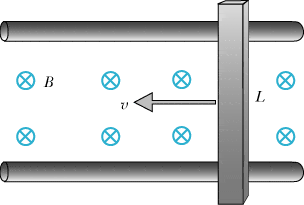# A neutral metal rod in a uniform magnetic field## Homework Statement

A neutral metal rod of length 0.35 m slides horizontally at a constant speed of 9 m/s on frictionless insulating rails through a region of uniform magnetic field of magnitude 0.6 tesla, directed into the page as shown in the diagram. Before answering the following questions, draw a diagram showing the polarization of the rod, and the direction of the Coulomb electric field inside the rod.

(A) After the initial transient, what is the magnitude of the net force on a mobile electron inside the rod?

(B) What is the magnitude of the electric force on a mobile electron inside the rod?

(C) What is the magnitude of the magnetic force on a mobile electron inside the rod?

(D) What is the magnitude of the potential difference across the rod?

Fmag + F(e) = F
Fmag = qBV
dV = EdL

## The Attempt at a Solution

(A) After the initial transient, what is the magnitude of the net force on a mobile electron inside the rod?
|Fnet|= 0 N (correct)
(B) What is the magnitude of the electric force on a mobile electron inside the rod?
|Fe|= 8.64E-19 N (incorrect)
(C) What is the magnitude of the magnetic force on a mobile electron inside the rod?
|Fmag|= 8.64E-19 N (correct)
(D) What is the magnitude of the potential difference across the rod?
|dV|= 1.89 V (correct)

Why am I getting the electric force wrong (all the others are correct)? If:

Fnet = 0, then:

Fmag + F(E) = 0, therefore:

F(E) = -Fmag, and when:

Fmag = 8.64E-19 N, then

F(E) = -8.64E-19 N, right?

How come it's not?

Last edited: